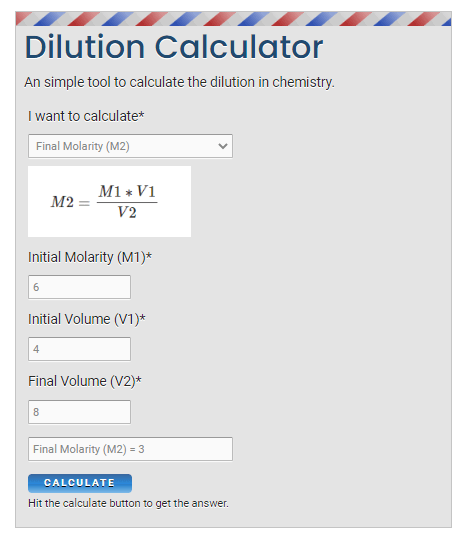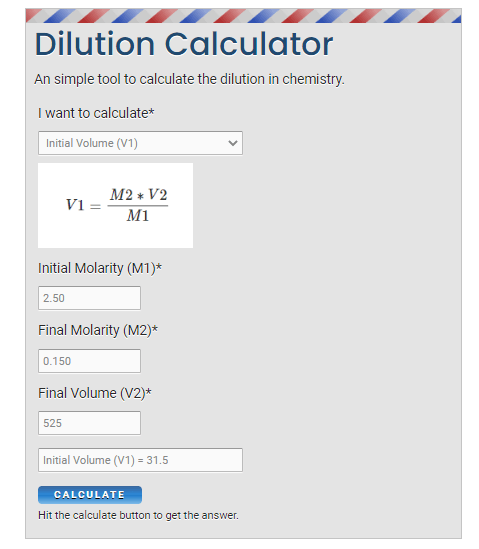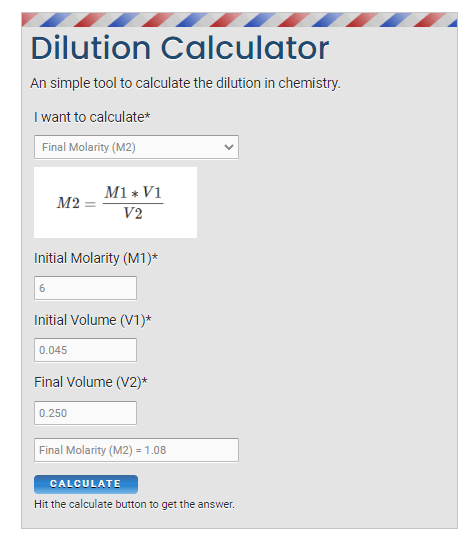# M1V1=M2V2 - Examples, Calculator, Uses, formula for dilution in chemistry?

Home > Chemistry > M1V1 = M2V2 Calculator and Examples

The equation (M1V1=M2V2) represents the dilution equation in chemistry. “Dilution is the process of decreasing the concentration of a solute in a solution, usually simply by mixing with more solvent like adding more water to the solution.”

## What does (M1V1 = M2V2) mean and where it is used in chemistry?

The equation (M1V1 = M2V2) is used to solve the problems related to dilution in chemistry where –

• M1 represents the molarity of an initial concentrated solution.
•  V1 represents the volume of the initial concentrated solution.
• M2 represents the molarity of the final diluted solution.
• V2 represents the volume of the final diluted solution.What is M1V1 = M2V2 Calculator?

An M1V1=M2V2 calculator is an online tool to determine the Molarity or Volume of the solution. The M1V1 = M2V2 calculator is also called the dilution calculator.

Dilution: It is a process in which the concentration of a solute in the solution is decreased by adding more solvent to the solution. In the dilution process, the concentration of the solution decreases while the volume of the whole solution increased.

The amount of dilute remains constant in the dilution process. (the number of moles is the same before and after dilution).

## Why is the (M1V1 = M2V2) formula used in the dilution problem, explain?

To understand this equation, we have to focus on the definition of Molarity. “Molarity is defined as the moles of a solute per liters of a solution”.

∴ Molarity = Moles of solute/liters of solution

We can also write it as –

⇒ Moles of solute = (Molarity) × (Liters of solution)

∴ Moles of solute = M × V      [∴ M represents molarity and V represents Volume]

In dilution process, the number of moles is the same before and after dilution. That means (M×V) remains same before and after concentration change.

So, according to this, we can write the initial and final conditions as –

M1V1 = M2V2

The above equation represents the dilution equation.

## Does M1V1 = M2V2 have to be in liters?

The short answer, it doesn’t matter whether should be M1V1 or M2V2 in liters or not.

What really matter is –

• The initial and final concentrations must have the same units.
• If your initial concentration is in moles per liter and final concentration should also have the same unit(moles per liter).
• Also, if the concentration is in moles per liter, then, it doesn’t necessary that the volume should also be in liters. But the thing is, the initial volume and final volume must have the same unit.
• Let’s say if V1 is in milliliters then V2 should also be in milliliters, and vice versa, if the given volume is in liters then another volume should also be in liters.

## How to solve dilution problems using (M1V1 = M2V2)?

It’s really easy, all you have to do is rearrange the formula(M1V1=M2V2) according to the question needs and find the value of the unknown one.

In most of the dilution problems, you have to ask to find either the concentration or volume in either the initial side or the final side of the equation.

 For example, If in a given problem, the value of M1 is x, for M2, it is y, and for V2, it is z. Now find the value of V1 =?

∴ We have, M1V1 = M2V2

Put the given values in the above equation-

⇒ xV1 = yz

That’s how simple it is to solve the problem of dilution using the equation M1V1 = M2V2.

## Examples of dilution problems using (M1V1 = M2V2)?

Let’s take some examples to understand the process of solving dilution problems using the equation (M1V1 = M2V2).

 Q1. A solution having a molarity of 6 M and a volume of 4 liters, after the dilution, the volume of the solution becomes 8 liters, then, calculate the molarity of the dilute solution? Answer – In the given problem, the initial molarity is 6 M, and the initial volume is 4 liters.So, we have, M1 = 6 and V1 = 4Also, it is given, that after the dilution, the volume of the solution becomes 8 liters.So, V2 = 8 Now we have to find the molarity of the dilute solution which means M2 =?Use the equation, M1V1 = M2V2∴ M2 = M1V1/V2= (6 × 4)/8= 3∴ So, the molarity of dilute solution (M2) is 3. Or use the M1V1 = M2V2 calculator to solve this problem –Q2. A solution having a molarity of 8 M and a volume of 4 liters. If 2 liters of solvent are added to the solution, calculate the molarity of the final solution? Answer = Given, M1 = 8, V1 = 4“If 2 liters of solvent are added to the solution”∴ V2 = V1 + 2= 4 + 2 V2 = 6 litersNow we have to find M2.Use the equation, M1V1 = M2V2∴ M2 = M1V1/V2= (8 × 4)/6= 16/3 ∴ M2 = 5.33 Or use the M1V1 = M2V2 calculator to solve this problem –Q3. How many mL of a 2.50 M NaOH solution are required to make 525 mL of a 0.150 M NaOH solution? Answer –In given problem, we have given, M1 = 2.50, M2 = 0.150, V2 = 525We have to find, V1 =? Use the equation, M1V1 = M2V2∴ V1 = M2V2/M1= (0.150 × 525)/2.50= 31.5∴ V1 = 31.5 mL Or use the M1V1 = M2V2 calculator to solve this problem –Q4. If 45.0 mL of a 6.00 M HCl solution is diluted to a final volume of 0.250 L, what is the final concentration? Answer –Given, M1 = 6, V1 = 45 mLAlso, the final volume (V2) is 0.250 L, we have to find, M2 =? We have one problem here, in the given question, V1 is in milliliters and V2 is in Liters, so, both have different units.According to the dilution equation concept –The initial and final volume must have same units.For example – If V1 is in liter then V2 must also be in liters.So, what we have to do is convert the milliliter unit to a liter.V1 = 45 mL = 0.045 LiterNow put these values in the dilution equation- ⇒ M1V1 = M2V2∴ M2 = M1V1/V2= (6 × 0.045)/0.250= 1.08∴ M2 = 1.08 M Or use the M1V1 = M2V2 calculator to solve this problem –## FAQ

### What does M1V1=M2V2 equation in chemistry represent?

We know, that M1V1=M2V2 represents the dilution equation, that M1 represents initial molarity, M2 represents final molarity, V1 represents initial volume and V2 denotes final volume.

### How can you find initial molarity (M1), initial volume (V1), final molarity (M2), and final volume (V2) using the M1V1=M2V2 equation?

The given equation is – M1V1=M2V2

Now to find the initial molarity (M1), initial volume (V1), final molarity (M2), and final volume (V2) use the formulas given below-Did you like it?### Vishal Goyal

My name is Vishal Goyal and I am the founder of Topblogtenz. I hold a degree in B.tech (Chemical Engineering) and have a strong passion for the life sciences and chemistry. As a highly qualified and experienced chemistry tutor with 4 years of experience, I possess a deep understanding of the unique challenges that students often encounter when attempting self-study in the field of chemistry. I have created this website as a comprehensive resource for those who are seeking guidance and support in their chemistry studies. I have brought together a team of experts, including experienced researchers, professors, and educators, to provide our readers with accurate and engaging information on a wide range of chemistry and science topics. Our goal is to make complex subjects like chemistry accessible and understandable for all. I hope you find the information and resources on our site helpful in your studies. Let's connect through LinkedIn: https://www.linkedin.com/in/vishal-goyal-2926a122b/

Share it...

## Connect with us

Email = [email protected]